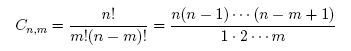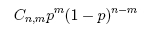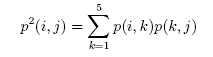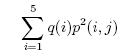# Yahtzee Solutions

1. Here it is easier to find the probability of not getting a six. Each time you roll, the probability of not getting a six is 5/6, thus the probability of not getting a six at all is (5/6)3 = 125/216. So the probability of getting at least one six is 1 - (125/216) = 91/216, or about 0.4213.

2. On the first roll you will fail to get a 1 or 6 with probability 4/6 = 2/3. The probability you fail twice is then (2/3)2 = 4/9, so you succeed with probability 1 - (4/9) = 5/9 = 0.5555.

3. On the first roll you will fail to get a 4 with probability 5/6 . The probability you fail twice is (5/6)2 = 25/36 so you succeed with probability 1 - (25/36) = 11/36 or about 0.3056. So the probability of succeeding in this case is lower than in the previous case. This is because here you are looking for an "inside" straight, so only one number works, while in the previous problem you were looking for an "outside" straight and had two numbers that would work.

4. The probability we do not roll a six in two tries with one die is (5/6)2, so the probability that we do not roll a 6 when we roll three dice twice each is (5/6)6 &asymp 0.3349. Therefore the probability of rolling at least three 6's is 1 - 0.3349 = 0.6651.

To finish with exactly four sixes we need to be successful (i.e. roll a six in two tries) with two dice and fail with the one other die. By the binomial distribution, this occurs with probability 3 · (11/36)2 · (25/36) &asymp 0.1945.

For a Yahtzee we must be successful with all 3 dice which occurs with probability (11/36)3 = 0.0285.

5. There are 6^2 = 36 possible outcomes when we re-roll the 1 and the 4. We first calculate the number of different outcomes that result in a particular hand and use this to determine the probability of each hand. For example, the probability of rolling a 2 and a 6 is 2/36 since we could roll the 2 and then the 6, or the 6 and then the 2. The probabilities of each of the hands are:

 Hand Number of Outcomes Probability Straight 6 - 2 2 2/36 = 1/18 Large 1 - 2 2 2/36 = 1/18 Large 6 - (not 2) 4 · 2 + 1 = 9 9/36 = 1/4 Small 2 - (not 1 or 6) 3 · 2 + 1 = 7 7/36 Small no 2 or 6 4^2 = 16 16/36 = 4/9 None

Note that a six paired with any other number can occur two ways, but a pair of sixes occurs in only one way. This is why the third and fourth rows have a plus one in the number of outcomes column.

Next, for each of the possible first roll outcomes we calculate the probability of getting a large straight or a small straight on the third roll. For the 6-2 and 1-2 hands we already have our large straight so we are done. For the 6 - (not 2) hand we re-roll the non-2 die. We have a 1/6 chance of getting a two and a large straight, and a 5/6 chance of not getting a 2 and having only a small straight. For the 2 - (not 1 or 6 hand) we will keep the 2,3,4,5 and re-roll one die, giving us a 1/3 chance at rolling a large straight and a 2/3 chance of having only a small straight. In the case where we rolled no 2 or 6 on the second roll, the situation for our third roll is the same as the situation for our second roll, so the above table gives the probabilities of each outcome.

Combining these results we see that the probability of a large straight is

1/18 + 1/18 + (1/4) · (1/6) + (7/36) · (1/3) + (4/9) · (1/18 + 1/18) &asymp 0.267.

The probability of a small straight (but not a large straight) is

(1/4) · (5/6) + (7/36) · (2/3) + (4/9) · (1/4 + 7/36) &asymp 0.535.

The probability of getting no straight at all is (4/9)2 &asymp 0.198.

If we re-roll only one die, then on our second roll we have a 1/6 chance of rolling a 2 and getting a large straight. We also have a 1/6 chance of getting a 6 and a small straight. In this case, on our third roll we have a 1/6 chance of rolling a 2 and getting a large straight. However, 2/3 of the time we get neither a 2 or a 6 on our first roll in which case our situation does not change. Therefore our chance of getting a large straight is

1/6 + (1/6) · (1/6) + (2/3) · (1/6) &asymp 0.306

The probability of getting only a small straight is

(1/6) · (5/6) + (2/3) · (1/6) = 0.25.

The chance of getting no straight is (2/3)2 = 4/9 &asymp 0.444. So re-rolling only the 4 gives you a slightly higher chance of getting the large straight, but a much lower probability of at least ending up with a small straight. If you only need a large straight then re-rolling just the four is the better strategy, but if a small straight would also be valuable then re-rolling the 1 and the 4 might be a better move.

6. The strategy we proposed for the two rolls game has the following expected score:Now for the three rolls game, using the same idea as in the two rolls game, we could use the following strategy: after the first roll: keep a 5 or a 6 and re-roll a 1, 2, 3, or 4. The expected score using this strategy is:(the last term in the sum comes from the fact that, if you re-roll after the first roll, then you are back in the situation of the two rolls game, so your expected score is 4.75, as before). So this strategy for the three rolls game has a better expected score than the strategy for the two rolls game.
7. Recall that the number of ways of picking m things out of n isA concrete example we will need in a minute isThere are 6^5 = 7776 outcomes for the first roll. The number of these that lead to the different hand patterns are as follows:

 Pattern Formula for Outcomes Total Outcomes 5 6 6 4 - 1 6 · C5,4 · 5 150 3 - 2 6 · C5,3 · 5 300 3 - 1 - 1 6 · C5,3 · 5 · 4 120 2 - 2 - 1 C6,2 · C5,2 · C3,2 · 4 1800 2 - 1 - 1 - 1 6 · C5,2 · 5 · 4 · 3 3600 1 - 1 - 1 - 1 - 1 6 · 5 · 4 · 3 · 2 720

To explain, 4-1 means four of one number and one of another. There are 6 values we can pick for the four of a kind, C5,4 ways of picking the dice that will show this number, and 5 values to assign to the remaining die. The hand pattern 2-2-1 is perhaps the trickiest. There are C6,2 ways of picking the two values that will appear twice, C5,2 ways of picking the two dice that will show the higher pair, C3,2 ways of picking the dice for the lower pair, and 4 values for the remaining die.

If we keep the most common number then the number of copies we have will be

 Copies, i 1 2 3 4 5 Outcomes 720 5400 1500 150 6 Probability, q(i) 0.092593 0.694444 0.19201 0.01929 0.000772

The binomial distribution tells us that the probability of getting m successes in n trials when the success probability is p isThe first factor gives the number of ways to pick the m trials on which success happens. The second gives the probability of any particular outcome with m successes and n-m failures. From this it follows that if we have i of a number before a roll then after the roll we will have i+k of that number with probabilityEvaluating these probabilities gives the following transition probability matrix

 from/to 1 2 3 4 5 1 625/1296 (125 · 4)/1296 (25 · 6)/1296 (5 · 4)/1296 1/1296 2 0 125/216 (25 · 3)/216 (5 · 3)/216 1/216 3 0 0 25/36 (5 · 2)/36 1/36 4 0 0 0 5/6 1/6 5 0 0 0 0 1

Expressed as decimals the matrix becomes

 from/to 1 2 3 4 5 1 0.482253 0.385802 0.115741 0.015432 0.000772 2 0 0.578704 0.347222 0.069444 0.00463 3 0 0 0.694444 0.277778 0.027778 4 0 0 0 0.833333 0.166667 5 0 0 0 0 1

To compute the probability of j of the given type of die after 2 rolls when we start with i of that type of die we square our transition matrix:In words, to go from i of a kind to j of a kind in two steps we must be at some state k after one step. Summing the probability of going from i to k and then from k to j over all possible k values gives the total probability of going from i to j. The result is

 from/to 1 2 3 4 5 1 0.232568 0.40932 0.270151 0.079244 0.008717 2 0 0.334898 0.442065 0.194509 0.028528 3 0 0 0.482253 0.424383 0.093364 4 0 0 0 0.694444 0.305556 5 0 0 0 0 1

To calculate the probability r(j) of ending up with j of a kind it remains to sumThis is the sum over all possible i of getting i of a kind on the first roll and then transitioning to j of a kind over the next two rolls, which gives the total probability of ending up with j of a kind using our strategy. This can also be obtained by multiplying the row vector with elements the q(i) with the matrix p2(i,j). Performing this computation yields

 copies, j 1 2 3 4 5 Probability, r(j) 0.021534 0.270468 0.424601 0.237294 0.045211

Thus the probability of a Yahtzee is roughly 1/22, while the chance of getting four of a kind is more than 1/4. With filling boxes in the upper section in mind, note that 28% of the time we will end up with only 2 or 1.

8. We can use the same techniques as for the previous problem, but now we must consider situations where after one roll we keep the dice of certain number, but after the next roll we end up with the most common number being something different.

First note that if we start with three or more of the same number than this type of situation can not occur; at most we could end up with two of some other number after our next roll. Therefore the bottom three rows of our transition matrix remain unchanged.

If we get all different numbers after one of our rolls then we are going to re-roll all of the dice (it would not affect our Yahtzee probability if instead we kept one die, why?). Therefore the elements of the first row of our new transition matrix are just the probabilities q(i) of getting i of a kind on our first roll.

For the second row, we begin by noting that if we are keeping two dice of some number then we can not get four or five of some different number on the next roll. Therefore the probabilities p(2,4) and p(2,5) are unchanged. However, it is possible that all three of the dice that we re-roll will be the same number (but different from our original number). Since there are five different numbers that we could roll, this occurs with probability 5 · (1/6)3 = (5/216) &asymp 0.023148. Therefore the new probability p(2,3) is (5/216) greater than the old probability, giving a value of (80/216) &asymp 0.37037. These rolls of three of a new kind of number were previously counted as transitions from two of a kind to two of a kind, so the probability p(2,2) must decrease by (5/216), giving a new value of (120/216) &asymp 0.55556. Therefore, our new transition matrix is

 from/to 1 2 3 4 5 1 0.092593 0.694444 0.19201 0.01929 0.000772 2 0 0.555556 0.37037 0.069444 0.00463 3 0 0 0.694444 0.277778 0.027778 4 0 0 0 0.833333 0.166667 5 0 0 0 0 1

In this problem we re-rolled if we had no matching dice. Therefore, before our first roll we are in the same situation as if we had rolled no matching dice on the previous turn. Therefore, taking the cube of the transition matrix, the first row will give us the probability of each outcome after three turns. The cubed transition matrix is

 from/to 1 2 3 4 5 1 0.000794 0.256011 0.452402 0.244765 0.046029 2 0 0.171468 0.435814 0.316144 0.076575 3 0 0 0.334898 0.487611 0.177491 4 0 0 0 0.578704 0.421296 5 0 0 0 0 1
Using this strategy we have a 4.6% chance of getting a Yahtzee, slightly higher than the Yahtzee probability for the simplified strategy in the previous problem. The probability of getting at least three of a kind is now just under 75%, as opposed to just under 71% in the previous problem.